[中文] Search Internet Search HelloDBA
Oracle Technology Site. email: fuyuncat@gmail.com  MSN: fuyuncat@hotmail.com# CBO Statistics data gathering and internal arithmetic analysis -- Calculating NDV and Density

[中文]

Author:  fuyuncat

Source:  www.HelloDBA.com

Date:  2010-01-18 06:23:13

Share to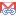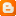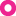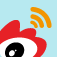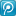## Calculating NDV and Density

Density is a import statistics data to affect the selectivity. It's normally derived from Number of Distinct Value (NDV), whose calculation is impacted by histogram with sampling.

### Frequency Histogram

When there is Frequency Histogram on the column, the calculation is simple, from the number of rows that the column value is not null, or says, the column rows SIZE.
Density = 1/(2*SIZE)

If the rows SIZE is from the sample data, means it's a sample size (SSZIE). It needs estimate the real SIZE first.
SIZE = SSIZE*100/PCT

And the formula of Density become,
Density = 1/(2*SIZE) = 1/(2*SSIZE*100/PCT) = PCT/(SSIZE*200)

### Height Balanced Histogram

With Height Balanced Histogram, the Density is calculated from Bucket sample size and popular value size, as well as their sum of sequal.
ssziesq=ssize1^2+ssize2^2...=∑SSIZEn^2
popcntsq=popcnt1^2+popcnt2^2...=∑POPCNTn^2

To choose which arithmetic, decided by Sample Number of Not null Values (SNNV) and smapling percent. For each range SNNV, if its scale to SDNV is in special limitation, Oracle will use a internal arithmetic named KKESDV scaling to estimate the NDV; Otherwise, if the estimated NNV and SNNV are in special limiatation, it will linear scale the NDV. If both kinds of limitation are exceeded, that means the histogram should be Height Balanced, need be converted to Frequency or adjust the sample percent.

I demo the density calculation of height balanced histogram by perl script.

SQL代码
1. HELLODBA.COM>host perl cal_height_balanced_dens.pl
2. ssize: 5401; ssizesq: 83601; popcnt: 0; popcntsq: 0; pct: 14.7058823529
3. sndv: 348.928852525687; snnv: 5401; nnv: 36726.8000001028
4. kksesdv scaling, density=0.00286532951289398

KKESDV Scaling arithmetic is a process to adjust the formula in loop. While we can get the linear scaling formula by replacing the factors with their own formula.
\$den = 1/(\$sndv*100/\$pct);
= 1/(\$ssize*((\$ssize-\$popcnt)/(\$ssizesq-\$popcntsq))*100/\$pct) # replace \$sndv by formula \$ssize*((\$ssize-\$popcnt)/(\$ssizesq-\$popcntsq))
= 1/(\$size*((\$ssize-\$popcnt)/(\$ssizesq-\$popcntsq)))           # \$size = \$ssize*100/\$pct
= (\$ssizesq-\$popcntsq)/(\$size*(\$ssize-\$popcnt))

### Without Histogram

When no histogram, the density is calculated from NDV.
Density=1/NDV

However, if stats data was analyzed from sample data (just get the SNDV), it needs scaling the NDV.

•   -If it's an unique column, NDV could be linear scaled.
NDV = ROUND(SNDV*100/PCT) => Density=1/ROUND(SNDV*100/PCT)
•   -If it's not an unique column, should check which scaling arithmetic it's suitable for.
•     If there is Height Balanced Histogram, the scaling will be same as Height Balanced (NDV = 1/Density).
•     If there is Frequency Histogram, will use the kkesdv scaling, the result must be same as SNDV;
•     If no histogram, the SNDV and SNNV is count() from the sample data, not calculatd from SSZIE and POPCNT.

Note, all of columns with sampling data will scale the NDV, but just those columns without calculated Density will calculate this data by 1/NDV. Since the histogram data is analyzed before scaling NDV, the Density may have calculated in the histogram analyzing process.

We can find the NDV scaling process from the Trace.

SQL代码
1. Scaling NDV of CREATED
2.  kkesdv_p: sndv=909,snnv=5401,nnv=46726,hind=49,slsv=0
3.  cht.count=284
4.  entries=76
5.  totcnt=75,popndv=0,lsv=0
6.  npndv=911
7.  kkesdv scaling or variant, ndv=911

### Checking

For those data calculated from sample data, oracle will finally double check them and revise them. For density, it will compare the calculated NDV and table row number (NROWS), if NDV larger than NROWS, it means it's unreasonable, should revise the density as 1/NROWS if it's calculated by 1/NDV.

Note: It will not calculate density&NDV for BLOB, CLOB, BFILE, CFILE, LONG, LONG RAW columns.

--- Fuyuncat  ---